Скачать презентацию CHAPTER 11 Factor markets and income distribution Mc

1139a8393da7e6147291f3e52de0e515.ppt

• Количество слайдов: 16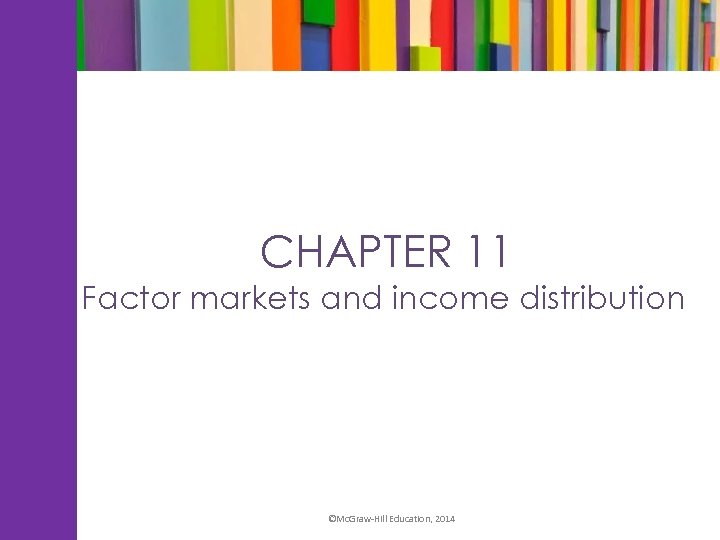CHAPTER 11 Factor markets and income distribution ©Mc. Graw-Hill Education, 2014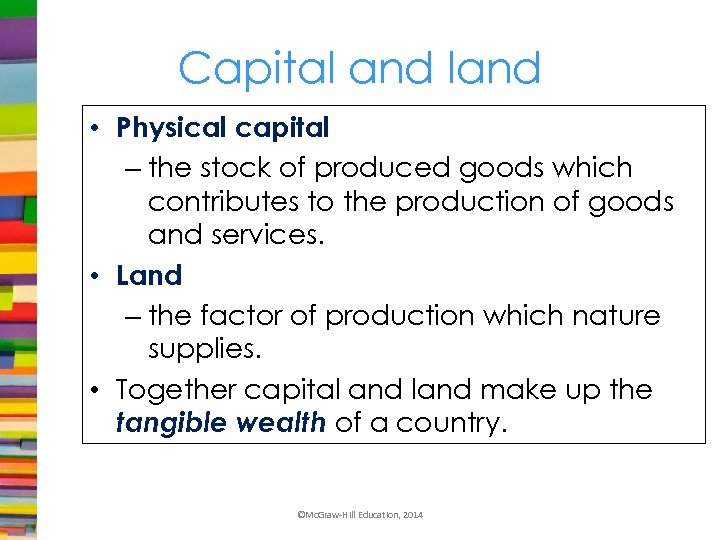Capital and land • Physical capital – the stock of produced goods which contributes to the production of goods and services. • Land – the factor of production which nature supplies. • Together capital and land make up the tangible wealth of a country. ©Mc. Graw-Hill Education, 2014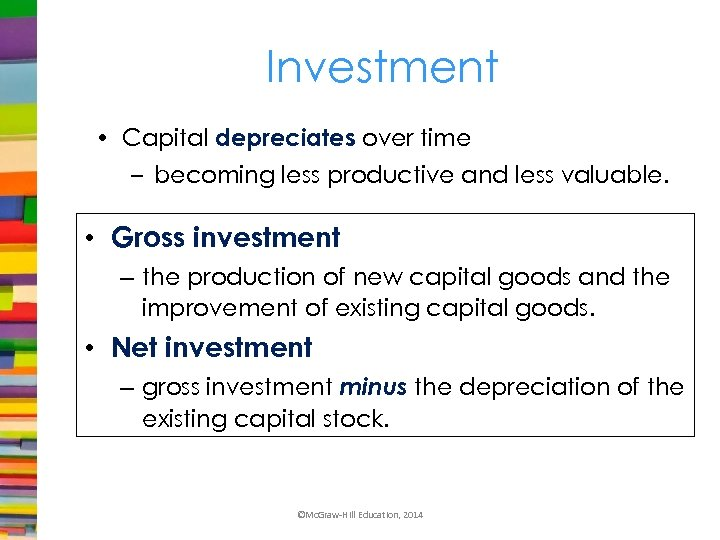Investment • Capital depreciates over time – becoming less productive and less valuable. • Gross investment – the production of new capital goods and the improvement of existing capital goods. • Net investment – gross investment minus the depreciation of the existing capital stock. ©Mc. Graw-Hill Education, 2014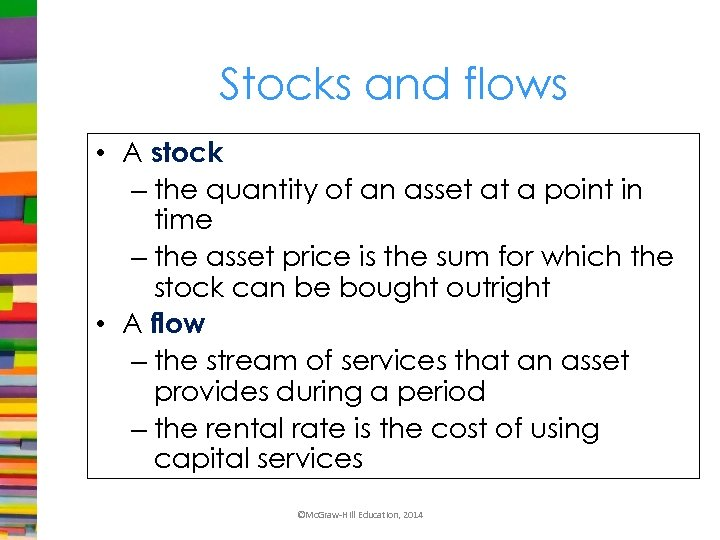Stocks and flows • A stock – the quantity of an asset at a point in time – the asset price is the sum for which the stock can be bought outright • A flow – the stream of services that an asset provides during a period – the rental rate is the cost of using capital services ©Mc. Graw-Hill Education, 2014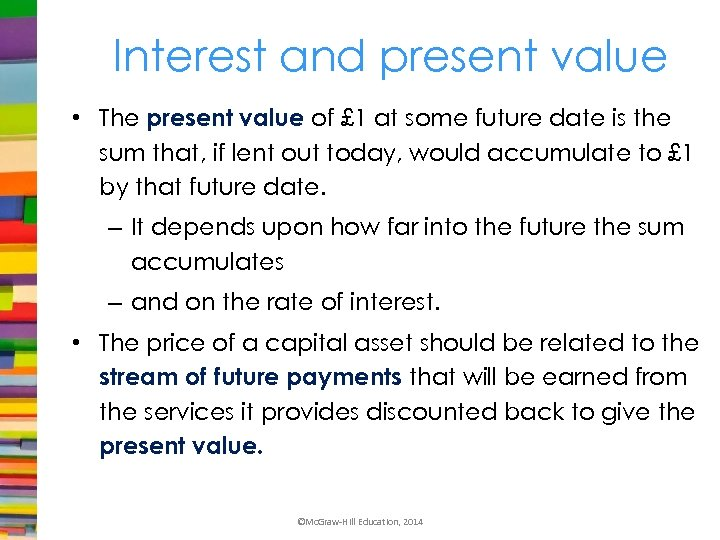Interest and present value • The present value of £ 1 at some future date is the sum that, if lent out today, would accumulate to £ 1 by that future date. – It depends upon how far into the future the sum accumulates – and on the rate of interest. • The price of a capital asset should be related to the stream of future payments that will be earned from the services it provides discounted back to give the present value. ©Mc. Graw-Hill Education, 2014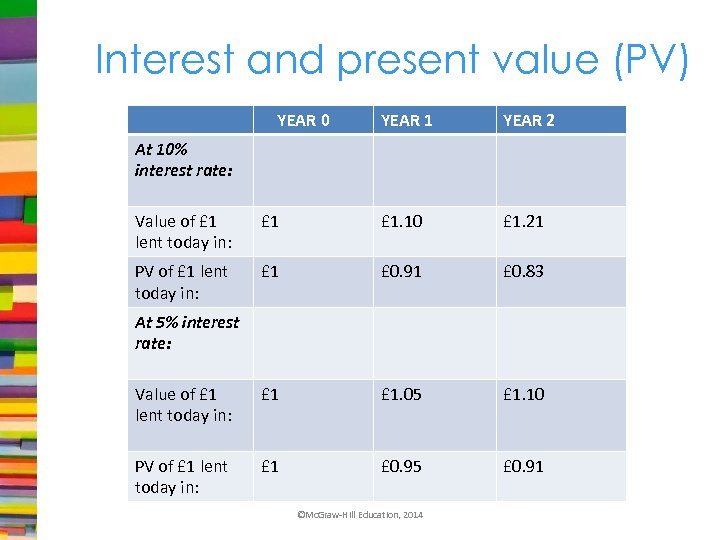Interest and present value (PV) YEAR 0 YEAR 1 YEAR 2 At 10% interest rate: Value of £ 1 lent today in: £ 1. 10 £ 1. 21 PV of £ 1 lent today in: £ 1 £ 0. 91 £ 0. 83 Value of £ 1 lent today in: £ 1. 05 £ 1. 10 PV of £ 1 lent today in: £ 1 £ 0. 95 £ 0. 91 At 5% interest rate: ©Mc. Graw-Hill Education, 2014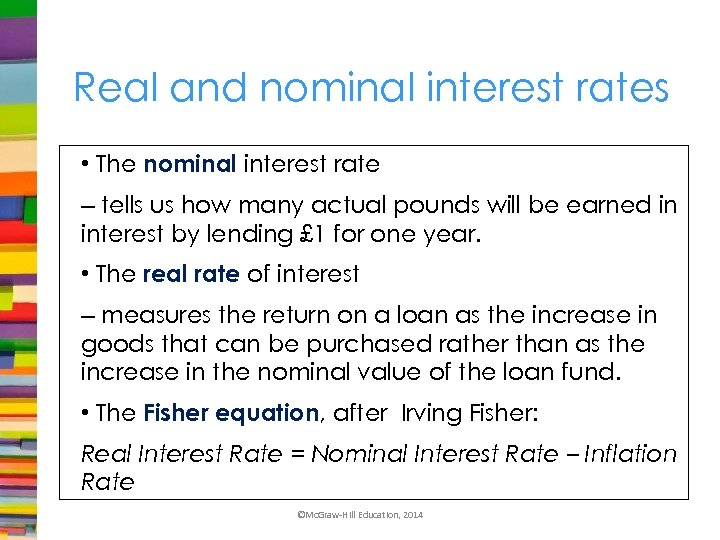Real and nominal interest rates • The nominal interest rate – tells us how many actual pounds will be earned in interest by lending £ 1 for one year. • The real rate of interest – measures the return on a loan as the increase in goods that can be purchased rather than as the increase in the nominal value of the loan fund. • The Fisher equation, after Irving Fisher: Real Interest Rate = Nominal Interest Rate – Inflation Rate ©Mc. Graw-Hill Education, 2014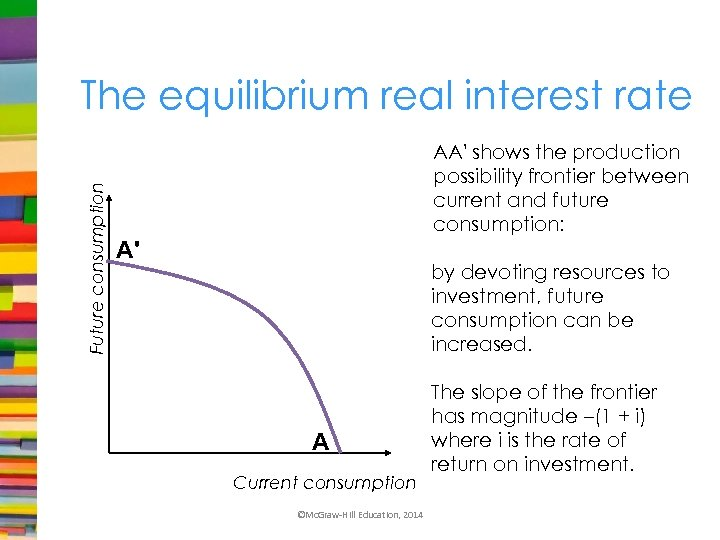Future consumption The equilibrium real interest rate AA' shows the production possibility frontier between current and future consumption: A' by devoting resources to investment, future consumption can be increased. A Current consumption ©Mc. Graw-Hill Education, 2014 The slope of the frontier has magnitude –(1 + i) where i is the rate of return on investment.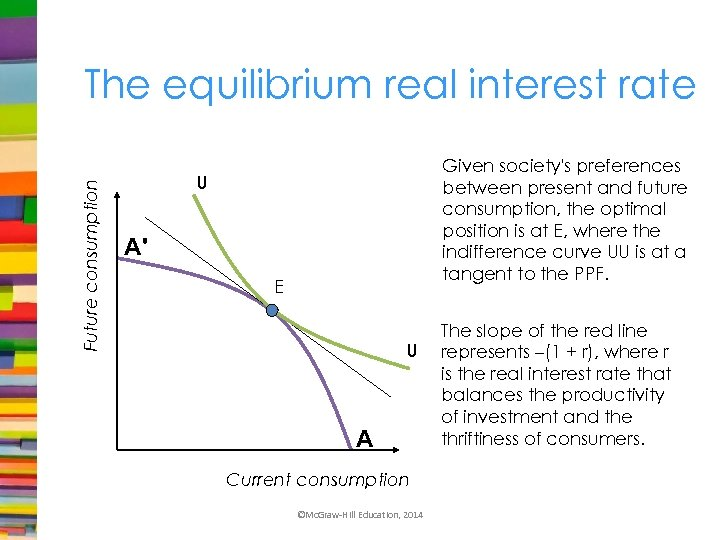Future consumption The equilibrium real interest rate Given society's preferences between present and future consumption, the optimal position is at E, where the indifference curve UU is at a tangent to the PPF. U A' E U A Current consumption ©Mc. Graw-Hill Education, 2014 The slope of the red line represents –(1 + r), where r is the real interest rate that balances the productivity of investment and the thriftiness of consumers.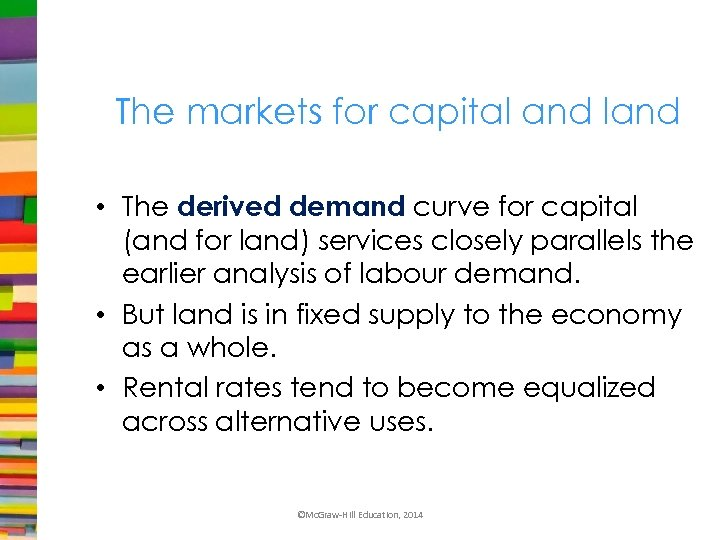The markets for capital and land • The derived demand curve for capital (and for land) services closely parallels the earlier analysis of labour demand. • But land is in fixed supply to the economy as a whole. • Rental rates tend to become equalized across alternative uses. ©Mc. Graw-Hill Education, 2014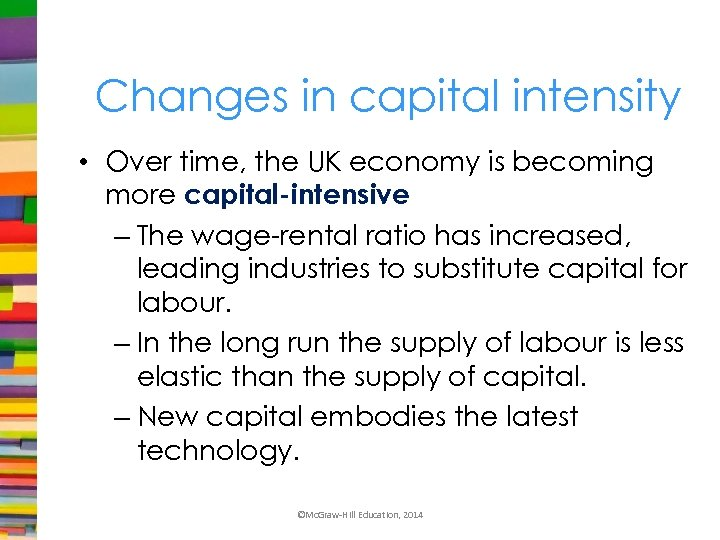Changes in capital intensity • Over time, the UK economy is becoming more capital-intensive – The wage-rental ratio has increased, leading industries to substitute capital for labour. – In the long run the supply of labour is less elastic than the supply of capital. – New capital embodies the latest technology. ©Mc. Graw-Hill Education, 2014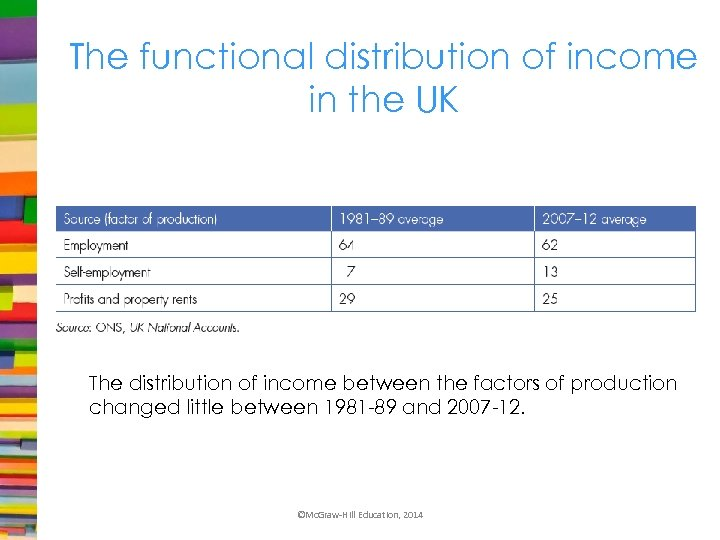The functional distribution of income in the UK The distribution of income between the factors of production changed little between 1981 -89 and 2007 -12. ©Mc. Graw-Hill Education, 2014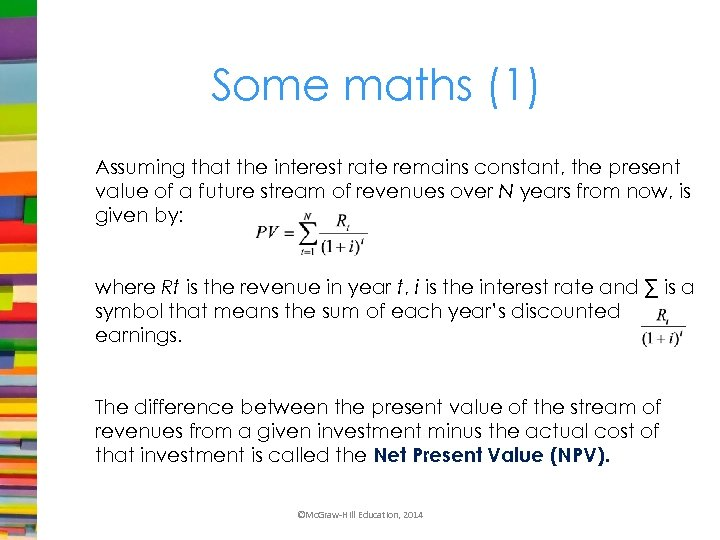Some maths (1) Assuming that the interest rate remains constant, the present value of a future stream of revenues over N years from now, is given by: where Rt is the revenue in year t, i is the interest rate and ∑ is a symbol that means the sum of each year’s discounted earnings. The difference between the present value of the stream of revenues from a given investment minus the actual cost of that investment is called the Net Present Value (NPV). ©Mc. Graw-Hill Education, 2014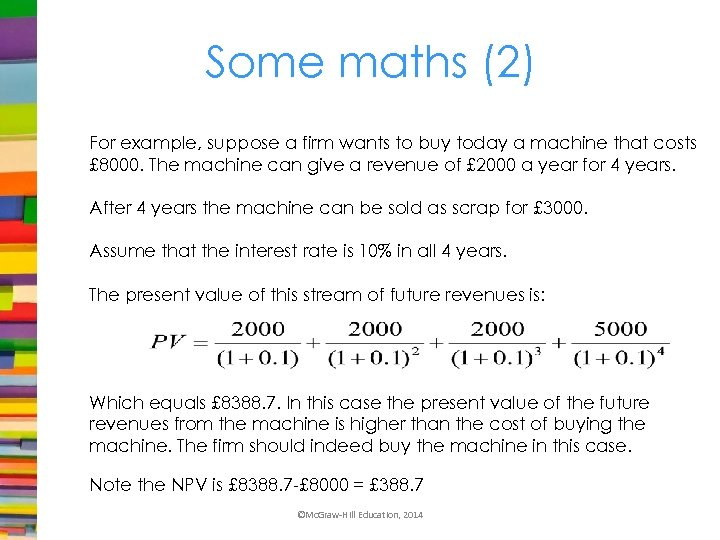Some maths (2) For example, suppose a firm wants to buy today a machine that costs £ 8000. The machine can give a revenue of £ 2000 a year for 4 years. After 4 years the machine can be sold as scrap for £ 3000. Assume that the interest rate is 10% in all 4 years. The present value of this stream of future revenues is: Which equals £ 8388. 7. In this case the present value of the future revenues from the machine is higher than the cost of buying the machine. The firm should indeed buy the machine in this case. Note the NPV is £ 8388. 7 -£ 8000 = £ 388. 7 ©Mc. Graw-Hill Education, 2014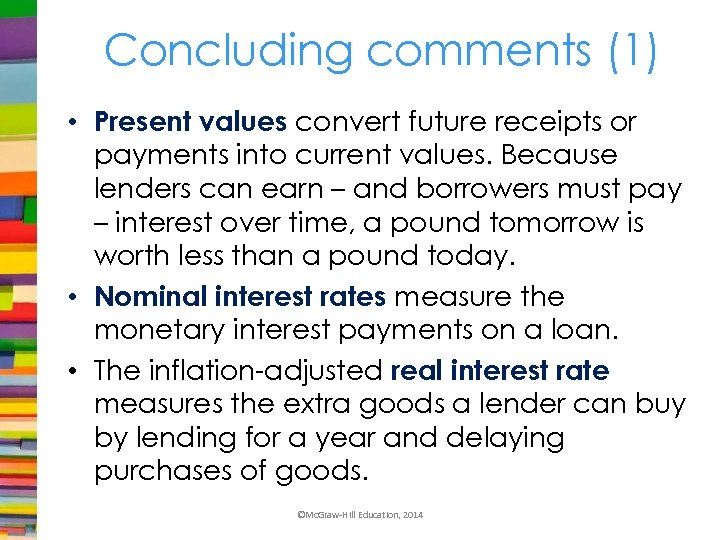Concluding comments (1) • Present values convert future receipts or payments into current values. Because lenders can earn – and borrowers must pay – interest over time, a pound tomorrow is worth less than a pound today. • Nominal interest rates measure the monetary interest payments on a loan. • The inflation-adjusted real interest rate measures the extra goods a lender can buy by lending for a year and delaying purchases of goods. ©Mc. Graw-Hill Education, 2014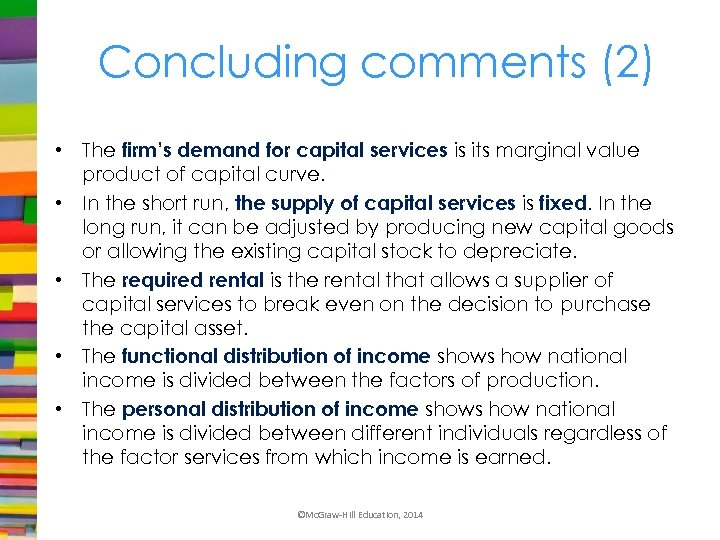Concluding comments (2) • The firm’s demand for capital services is its marginal value product of capital curve. • In the short run, the supply of capital services is fixed. In the long run, it can be adjusted by producing new capital goods or allowing the existing capital stock to depreciate. • The required rental is the rental that allows a supplier of capital services to break even on the decision to purchase the capital asset. • The functional distribution of income shows how national income is divided between the factors of production. • The personal distribution of income shows how national income is divided between different individuals regardless of the factor services from which income is earned. ©Mc. Graw-Hill Education, 2014